# High School Chemistry : Help with Molecular Formulas

## Example Questions

### Example Question #1 : Help With Molecular Formulas

Choose the correct chemical formula for sodium sulfate.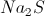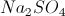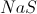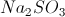Explanation:

Sulfate is a polyatomic ion that is composed of one sulfur atom and four oxygen atoms. The ion has a net charge of.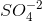Since sodium ions have a charge of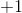, we must use two atoms of sodium in order to result in a neutrally charged compound.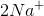This makes the neutral chemical compound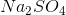.

### Example Question #2 : Help With Molecular Formulas

An unknown compound has the following composition:

40% carbon

6.7% hydrogen

53.3% oxygen

If the molecular formula for this compound is six times as heavy as the empirical formula, what is the molecular formula for the compound?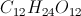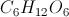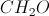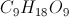Explanation:

Since we know the percentages by mass, we can convert them into grams by imagining a 100-gram sample of the mystery compound. At that point, we can determine how many moles are present for each element: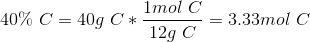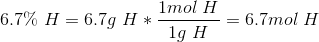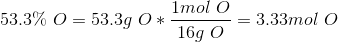By dividing each molar amount by the smallest molar amount (in this case 3.33), we can find the elemental ratios.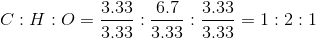This means that the empirical formula for this compound is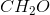.

Since we were told that the molecular formula is six times as heavy as the empirical formula, we need to multiply each elemental amount in the empirical formula by six. After doing this, we find that the molecular formula is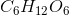.

### Example Question #3 : Help With Molecular Formulas

What specific functional group is created when a carbon atom forms a double bond with an oxygen atom and a single bond with an -OH group?

alcohol

Ester

Carboxyl group

Ether

Carbonyl group

Carboxyl group

Explanation:

Carboxylic acids are formed by a carbon atom with a double bond to an oxygen atom, as well as an -oh substituent. This leaves one remaining bond for the carbon atom, allowing it to bind to a larger molecular component. The name for a carboxylic acid bound to a larger molecule is a "carboxyl group."

Carbonyl groups are formed when a carbon atom forms a doubl bond with an oxygen atom and any two other substituents. Carboxylic acids, aldehydes, ketones, esters, and amides are all carbonyl functional groups.

An ester is formed when a carbon forms a double bond with an oxygen and a single bond with a second oxygen atom. The second oxygen is generally incorporated into the backbone structure of the molecule, rather than as a single substituent to the carbon.

An ether is formed by an oxygen atom bound to two other atoms (usually carbons).

### Example Question #4 : Help With Molecular Formulas

Which of the following options contains only compounds with covalent bonds?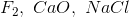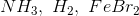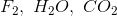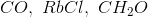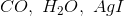Explanation:

Ionic bonds generally occur between metal and nonmetal ions, while covalent bonds usually occur between two nonmetal atoms. The compounds containing sodium, iron, silver, calcium, and rubidium will all contain ionic bonds involving these elements.

The answeris the only answer without any metal atoms, indicating that the bonds in these molecules will be covalent.

### Example Question #1 : Help With Molecular Formulas

Which of these molecules will not demonstrate hydrogen bonding?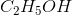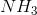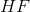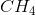Explanation:

Hydrogen bonds are present in molecules in which hydrogen atoms are bound to highly electronegative atoms, namely oxygen, fluorine, or nitrogen. These bonds are extremely polar, resulting in a partial positive charge on the hydrogen atoms and a partial negative charge on the more electronegative atom.

Of the given answer options, methane (), is the only one that does not involve a hydrogen atom bound to an oxygen, nitrogen, or fluorine. It cannot demonstrate hydrogen bonding.

Note that glucose (), as well as most other sugars, contain aldehyde or hydroxyl groups. These consist of -OH bonds, which allow for hydrogen bonding.

### All High School Chemistry Resources